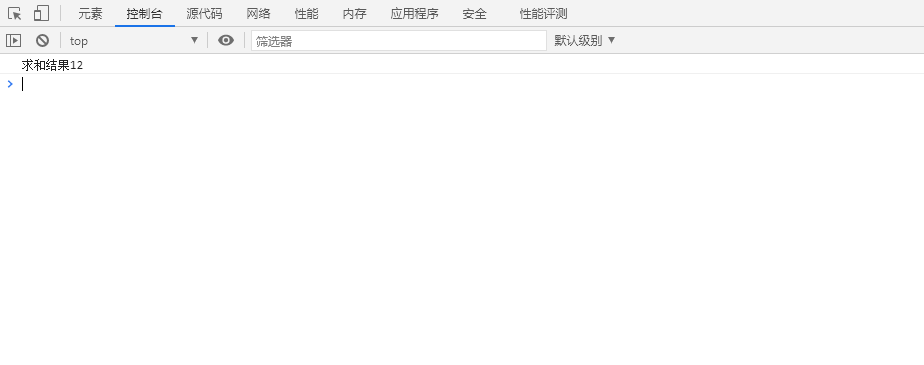# 第三次作业

<html>

<body>
<script>
//输入方式
var num1
num1 = parseInt(prompt("请输入第一个要进行运算的数据"))
var num2
num2 = parseInt(prompt("请输入第二个要进行运算的数据"))
var sum = num1 + num2;
//输出方式
document.write("求和"+sum);
//在控制台输出信息
console.log("求和结果"+sum)
</script>
</body>